## Statistics

Notations:
p.s. – period sequence
fano p.s. – one of 105 p.s. of smooth Fano 3-folds
good p.s. – p.s. s.t. P_0(D) has integer roots, where L = sum_{i=0}^r t^i P_i(D) is the diff.op. annihilating p.s.
bad p.s. – not good p.s.

LP – Laurent polynomial.
fano LP – Laurent polynomial for which RG coincides with one of 105 fano p.s.

Everywhere below we put a binomial condition (of Coates-Corti-Galkin-Golyshev-Przjyalkowski-Usnich-etc) on the edges.

MP – Minkowski Laurent polynomial
PP – (Coates-)Przyjalkowski Laurent polynomial (binomial on edges, zero everywhere else)

This is the statistics of reflexive 3-polytopes.

18 are smooth.

100 (= 18+82) are terminal. They give rise to 100 LP and 62 good p.s.

899 (= 18+82+799) has no integer points in interior of facets.

Each one gives rise to a unique MP (which coincides with PP).

712 of these MPs are fano LP – they give rise to 92 fano p.s.

92 = 98-6, where 98 = 105-7.
The 7 that didn’t appear among MP p.s.’s are:
V_2 [bottom degree],
its double cover B_1 [bottom degree in rho=1, r=2],
2.1, 2.2, 2.3 [bottom degree in rho=2],
P^1 x S_2, P^1 x S_1 [top Picard rank].

The extra 6 Fano (p.s.) that didn’t appear are:
V_4 (15), V_6 (19), V_8 (5), V_{10} (9), V_{12} (7) [next bottom degree in rho=1,r=1);
2.4 (49) [next bottom degree in rho=2].

So they lie at the bottom of the list, just over the non-appearing 5.

1051 polytopes has exactly one integer point in the respective interior of the facets (i.e. not origin, vertex, and not on the edge).

The facet that contains the extra integer point is then on of famous 16 reflexive 2-polytopes,
so 1051 polytopes fall into 16 classes.

Number of polytopes in each class is as follows (total 1051):
[20, 24, 125, 50, 75, 196, 22, 86, 111, 74, 64, 112, 19, 42, 23, 8]

Number of fano LP in each class is as follows (total 1055):
[0, 20, 0, 47, 61, 187, 27, 123, 195, 140, 56, 109, 17, 42, 23, 8]
which is
[0,17+3,0,44+3,61,187,16+11,71+52,107+88,70+70,56,109,17,42,23,8]

Our enumeration and “fano” values for the extra coefficient are as follows:

number – associated vertex Laurent polynomial – class – #poly – values [number of appearances]

0 – x+y+1/x/y – P^2 – 20 – nothing
1 – y + x/y + 1/x/y – Q – 24 – 0 , 4 
2 – x+y+xy+1/x/y – S_8 – 125 – nothing
3 – x+y+1/x+1/y – Q – 50 – 0 , 4 
4 – y+x+x/y+1/x/y – S_7 – 75 – 1 
5 – x+y+1/x+1/y+xy – S_7 – 196 – 1 
6 – y/x+1/x/y+x^2/y – S_6 – 22 – 2 , 3 
7 – y+y/x+1/x/y+x/y – S_6 – 86 – 2 , 3 
8 – y+x+1/x+x/y+1/x/y – S_6 – 111 – 2 , 3 
9 – x+y+x/y+1/x+1/y+y/x – S_6 – 74 – 2 , 3 
10- x+y/x+1/x/y+x^2/y – S_5 – 64 – 3 
11- x+y+y/x+x/y+1/x+1/y+1/x/y – S_5 – 112 – 3 
12- y+x+1/x+x^2/y+1/x^2/y – S_4 – 19 – 4 
13- y+y/x+1/x/y+x^2/y – S_4 – 42 – 4 
14- xy+y/x+x/y+1/x/y – S_4 – 23 – 4 
15- 1/x/y+x^2/y+y^2/x – S_3 – 8 – 6 

Of course everything is extremal and Hodge-Tate,
but some are not-Minkowski, and not even SCR-equivalent (using only surface mutations of the respective facet) to any Minkowski
(in particular examples with class Q and coefficient a=4,
also those in Q with coeff a=0 are not lattice Minkowski, but Minkowski).
1-puzzles

### One Comment

1.Sergey says:

So we know 1051 polytopes with 1 interior facet point produce 1055 fano LPs

How many non-fano LPs they produce if we assume locality and allow to assign the admissible coefficient(s) rather than matching fano p.s.?

Total numbers of non-fano LPs produced is 218, they fall into different classes as follows
[0, 28, 0, 53, 14, 9, 17, 49, 27, 8, 8, 3, 2, 0, 0, 0]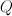$Q$ – 0, 4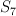$S_7$ – 7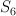$S_6$ – 2, 3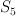$S_5$ – 3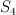$S_4$ – 4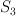$S_3$ – 6

Case #: number of non-fano LPs

Case 1: 28
a=0: 7(=24-17) non-fano LP
a=4: 21(=24-3) non-fano LP

Case 3: 53
a=0: 6(=50-44)
a=4: 47(=50-3)

Case 4: 14(=75-61)
Case 5: 9(=196=187)

Case 6: 17
a=2: 6(=22-16)
a=3: 11(=22-11)

Case 7: 49
a=2: 15(=86-71)
a=3: 34(=86-52)

Case 8: 27
a=2: 4(=111-107)
a=3: 23(=111-88)

Case 9: 8
a=2: 4(=74-70)
a=3: 4(=74-70)
It seems 4 bad cases are the same for a=2 and a=3,
can we find any explanation?

Case 10: 8(=64-56)
Case 11: 3(=112-109)

Case 12: 2(=19-17)
Case 13: 0(=42-42)
Case 14: 0(=23-23)

Case 15: 0(=8-8)